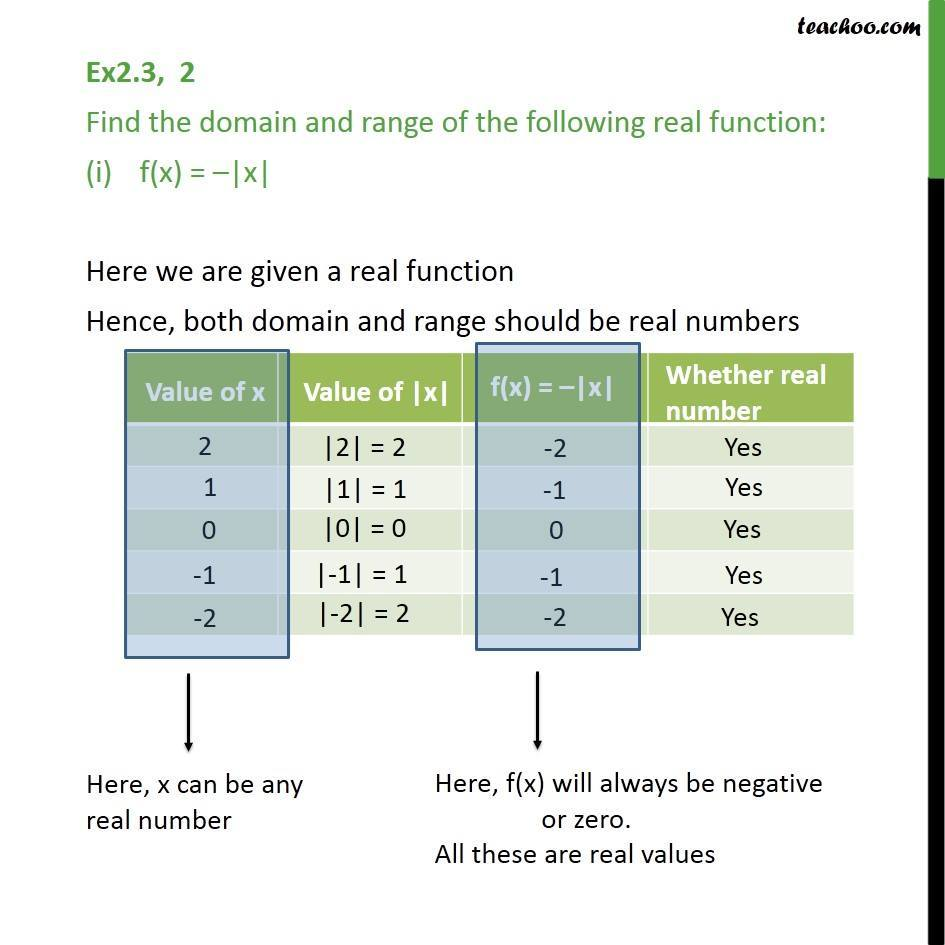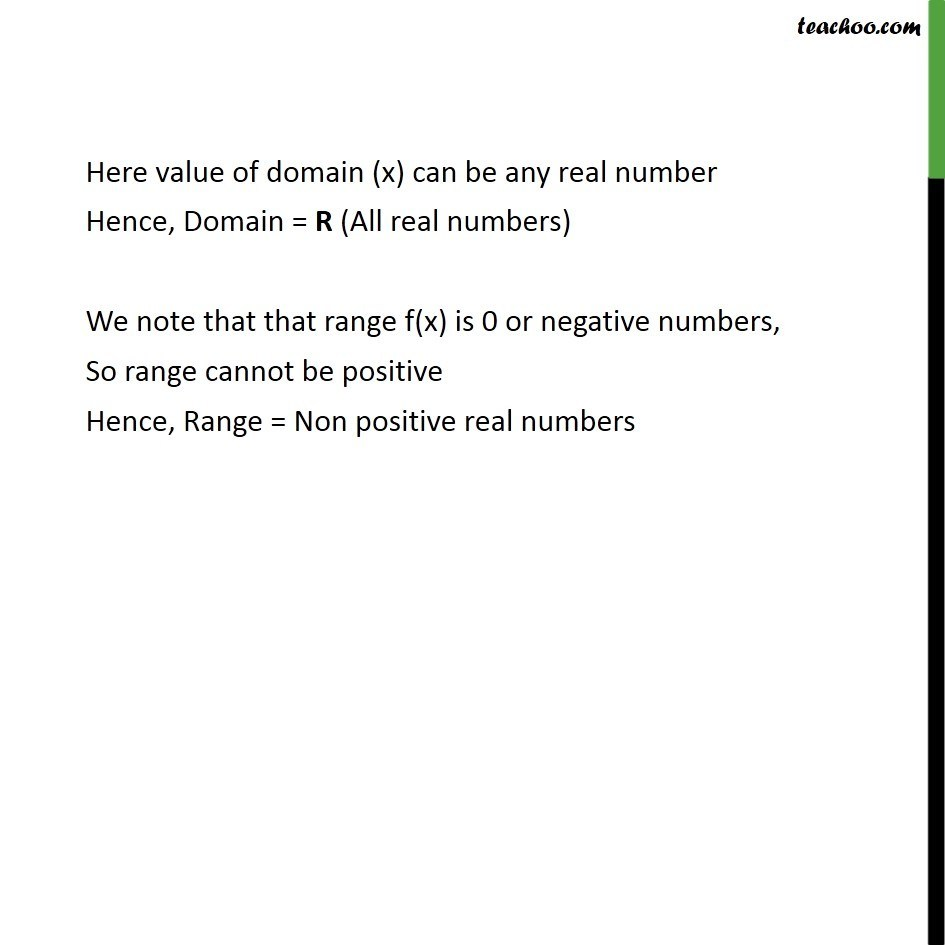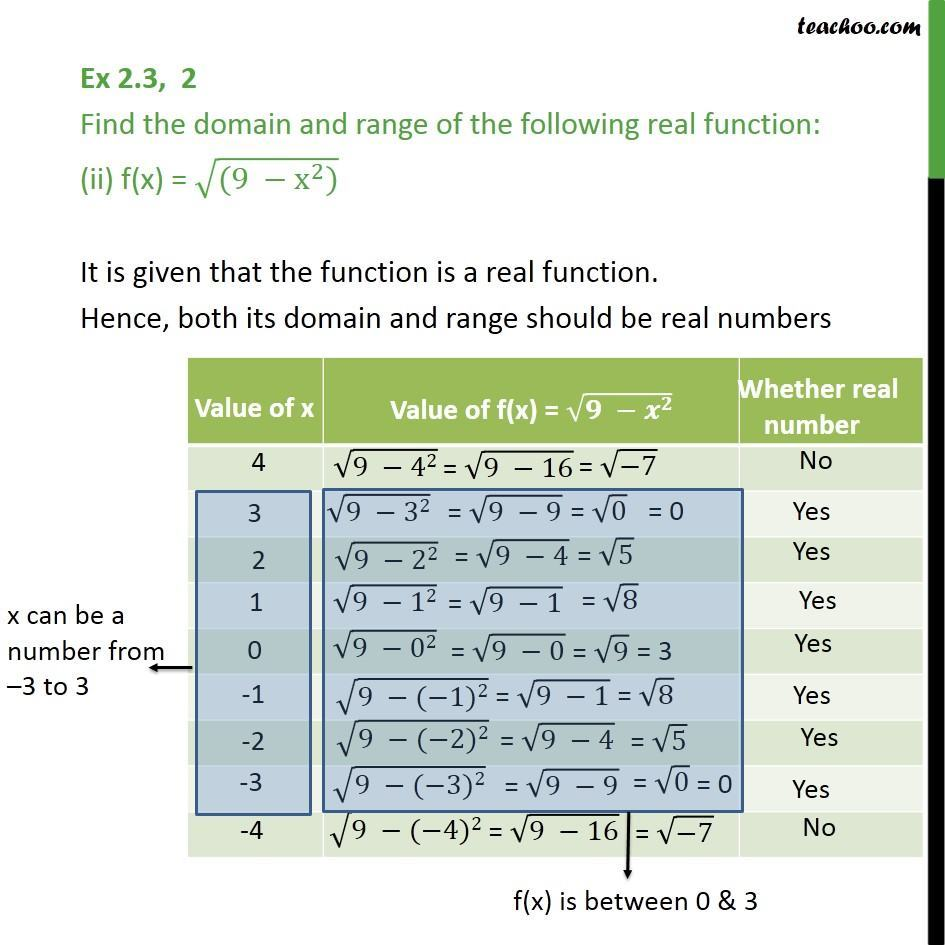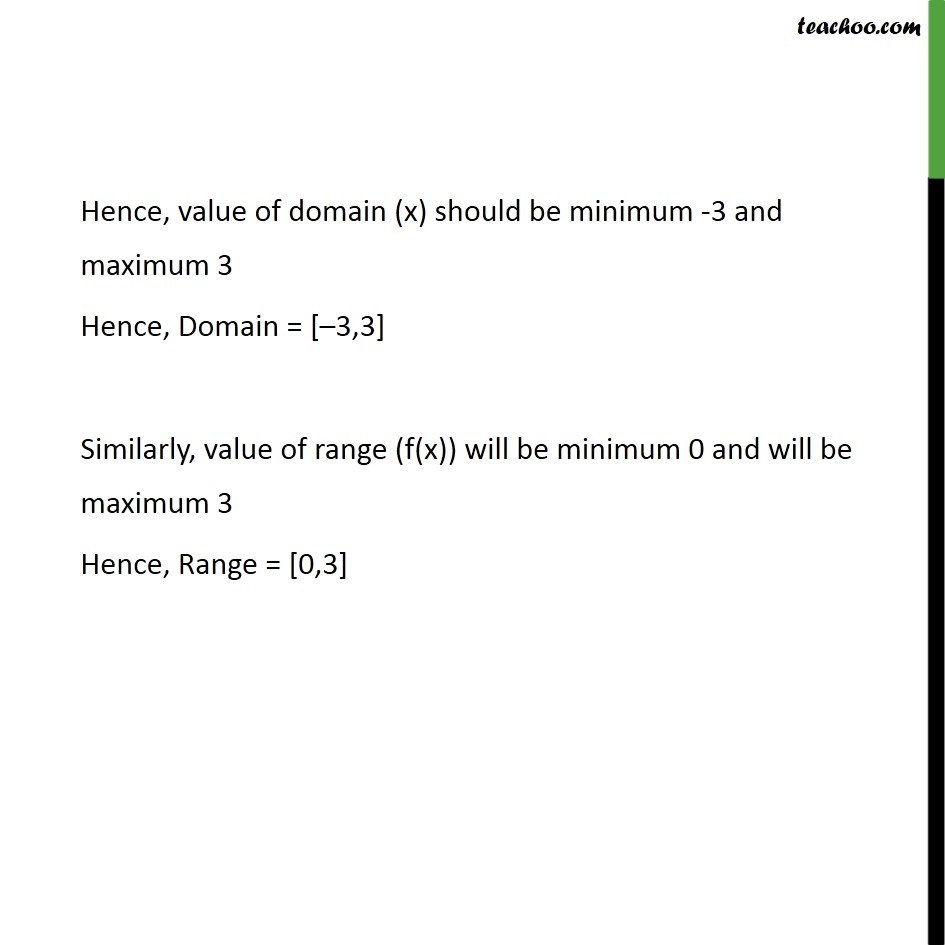1. Chapter 2 Class 11 Relations and Functions
2. Serial order wise
3. Ex 2.3

Transcript

Ex2.3, 2 Find the domain and range of the following real function: f(x) = –|x| Here we are given a real function Hence, both domain and range should be real numbers Here value of domain (x) can be any real number Hence, Domain = R (All real numbers) We note that that range f(x) is 0 or negative numbers, So range cannot be positive Hence, Range = Non positive real numbers Ex 2.3, 2 Find the domain and range of the following real function: (ii) f(x) = √((9 −x^2)) It is given that the function is a real function. Hence, both its domain and range should be real numbers Hence, value of domain (x) should be minimum -3 and maximum 3 Hence, Domain = [–3,3] Similarly, value of range (f(x)) will be minimum 0 and will be maximum 3 Hence, Range = [0,3]

Ex 2.3